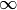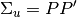# statsmodels.tsa.vector_ar.var_model.VARResults¶

class statsmodels.tsa.vector_ar.var_model.VARResults(endog, endog_lagged, params, sigma_u, lag_order, model=None, trend='c', names=None, dates=None)[source]

Estimate VAR(p) process with fixed number of lags

Parameters: endog : array endog_lagged : array params : array sigma_u : array lag_order : int model : VAR model instance trend : str {‘nc’, ‘c’, ‘ct’} names : array-like List of names of the endogenous variables in order of appearance in endog. dates Attributes aic bic bse coefs : ndarray (p x K x K) Estimated A_i matrices, A_i = coefs[i-1] cov_params dates detomega df_model : int df_resid : int endog endog_lagged fittedvalues fpe intercept info_criteria k_ar : int k_trend : int llf model names neqs : int Number of variables (equations) nobs : int n_totobs : int params k_ar : int Order of VAR process params : ndarray (Kp + 1) x K A_i matrices and intercept in stacked form [int A_1 ... A_p] pvalues names : list variables names resid roots : array The roots of the VAR process are the solution to (I - coefs*z - coefs*z**2 ... - coefs[p-1]*z**k_ar) = 0. Note that the inverse roots are returned, and stability requires that the roots lie outside the unit circle. sigma_u : ndarray (K x K) Estimate of white noise process variance Var[u_t] sigma_u_mle stderr trenorder tvalues y : ys_lagged

Methods

 acf([nlags]) Compute theoretical autocovariance function acorr([nlags]) Compute theoretical autocorrelation function bse() Standard errors of coefficients, reshaped to match in size cov_params() Estimated variance-covariance of model coefficients cov_ybar() Asymptotically consistent estimate of covariance of the sample mean detomega() Return determinant of white noise covariance with degrees of freedom fevd([periods, var_decomp]) Compute forecast error variance decomposition (“fevd”) fittedvalues() The predicted insample values of the response variables of the model. forecast(y, steps) Produce linear minimum MSE forecasts for desired number of steps forecast_cov([steps]) Compute forecast covariance matrices for desired number of steps forecast_interval(y, steps[, alpha]) Construct forecast interval estimates assuming the y are Gaussian get_eq_index(name) Return integer position of requested equation name info_criteria() information criteria for lagorder selection irf([periods, var_decomp, var_order]) Analyze impulse responses to shocks in system irf_errband_mc([orth, repl, T, signif, ...]) Compute Monte Carlo integrated error bands assuming normally irf_resim([orth, repl, T, seed, burn, cum]) Simulates impulse response function, returning an array of simulations. is_stable([verbose]) Determine stability based on model coefficients llf() Compute VAR(p) loglikelihood long_run_effects() Compute long-run effect of unit impulse ma_rep([maxn]) Compute MA() coefficient matrices mean() Mean of stable process mse(steps) Compute theoretical forecast error variance matrices orth_ma_rep([maxn, P]) Compute Orthogonalized MA coefficient matrices using P matrix such that. plot() Plot input time series plot_acorr([nlags, linewidth]) Plot theoretical autocorrelation function plot_forecast(steps[, alpha, plot_stderr]) Plot forecast plot_sample_acorr([nlags, linewidth]) Plot theoretical autocorrelation function plotsim([steps]) Plot a simulation from the VAR(p) process for the desired number of pvalues() Two-sided p-values for model coefficients from Student t-distribution reorder(order) Reorder variables for structural specification resid() Residuals of response variable resulting from estimated coefficients resid_acorr([nlags]) Compute sample autocorrelation (including lag 0) resid_acov([nlags]) Compute centered sample autocovariance (including lag 0) resid_corr() Centered residual correlation matrix roots() sample_acorr([nlags]) sample_acov([nlags]) sigma_u_mle() (Biased) maximum likelihood estimate of noise process covariance stderr() Standard errors of coefficients, reshaped to match in size summary() Compute console output summary of estimates test_causality(equation, variables[, kind, ...]) Compute test statistic for null hypothesis of Granger-noncausality, test_normality([signif, verbose]) Test assumption of normal-distributed errors using Jarque-Bera-style test_whiteness([nlags, plot, linewidth]) Test white noise assumption. tvalues() Compute t-statistics.

Attributes

 aic Akaike information criterion bic Bayesian a.k.a. df_model Number of estimated parameters, including the intercept / trends df_resid Number of observations minus number of estimated parameters fpe Final Prediction Error (FPE) hqic Hannan-Quinn criterion